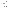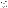# Math Stumper: Use Two Squares to Make Separate Pens for Nine Pigs

A word problem often involves computational strategy or strategies. In early elementary school years, word problems will generally focus on addition, subtraction, multiplication, and division. Word problems generally require specific steps to solve them.

Problem-solving, by contrast, differs in that there may be two or three steps to solve the problem and there also may be a variety of approaches that are accurate. Such problems are called math stumpers because they are somewhat open-ended and there are a few different strategies that students can use to solve the problem.

The math stumper below requires students to use two squares to make separate pens for nine pigs.

01
of 02

## The Problem and Solution9 Pigs Stumper.  Deb Russell

This section contains two worksheets: the first page shows nine pigs lined up in three rows of three. It will likely seem impossible to your students to use two squares to provide nine separate pens: one for each pig.

But to solve this stumper, students need to think outside the box—literally. Since you are requiring students to create nine pens for the pigs with two boxes, students will almost certainly think they need to use more and smaller boxes (or squares) to provide each pig with a separate pen. But that isn't the case.

The second page of the PDF in this section shows the solution. You use two boxes with one tipped on its side (like a diamond) and another square placed perpendicularly within that square. The outside box creates eight triangle-shaped squares for eight pigs. The ninth pig gets a larger, and square, pen within its own box. The problem never said all the pens had to be square or in the same shape.

02
of 02

## Making Problem Solving Fun9 Pigs Stumper Solution.  Deb Russell

The main reason for learning about math is to become a better problem solver. There are a couple of things students need to do when solving problems. They should ask exactly what type of information is being asked for. Then they need to determine all the information that is being provided in the question.

In the nine-pig problem, students were shown a picture of nine pigs and asked to provide pens for each using only two boxes. To solve the pig-pen problem, explain to students that they should think of themselves as math detectives. That means—as the fictional detective Sherlock Holmes might have pointed out—eliminating all extraneous noise and unnecessary clutter and focusing on the facts as presented.

You can vary or extend this exercise by asking students to put nine pigs into four pens so that there is an odd number of pigs in each pen. Remind students that this problem, as the previous one, does not specify the shape of the pens, so they might well start with square pens. The solution here is that the pens are joined. Four pens on the outside each contain an odd number of pigs (one), and a pen is placed in the middle of the four pens (so it's "inside the pens"), and it contains an odd number of pigs (five).

Format
mla apa chicago
Your Citation
Russell, Deb. "Math Stumper: Use Two Squares to Make Separate Pens for Nine Pigs." ThoughtCo, Aug. 26, 2020, thoughtco.com/9-pigs-math-problem-2312632. Russell, Deb. (2020, August 26). Math Stumper: Use Two Squares to Make Separate Pens for Nine Pigs. Retrieved from https://www.thoughtco.com/9-pigs-math-problem-2312632 Russell, Deb. "Math Stumper: Use Two Squares to Make Separate Pens for Nine Pigs." ThoughtCo. https://www.thoughtco.com/9-pigs-math-problem-2312632 (accessed April 18, 2021).